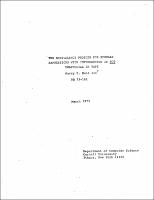## The Equivalence Problem for Regular Expressions with Intersection is Not Polynomial in Tape##### Abstract
We investigate the complexity of several predicates on regular sets. In particular, we show: 1) the equivalence and emptiness problem for regular expressions using only the operators -, $\cup$, ., and $\cap$ are p-complete. 2) the emptiness problem for regular expressions using the operators -, $\cup$, ., $\cap$ and * is tape-hard; 3) the emptiness problem for regular expressions using the operators -, $\cup$, ., $\cap$ and 2 is tape-hard; 4) the equivalence problem for regular expressions using the operators -, $\cup$, ., $\cap$ and * is not polynomial in tape; and 5) the equivalence problem for regular expressions using the operators -, $\cup$, ., $\cap$ and 2 requires exponential time.
1973-03
##### Publisher
Cornell University
##### Keywords
computer science; technical report
##### Previously Published As
http://techreports.library.cornell.edu:8081/Dienst/UI/1.0/Display/cul.cs/TR73-161
technical report